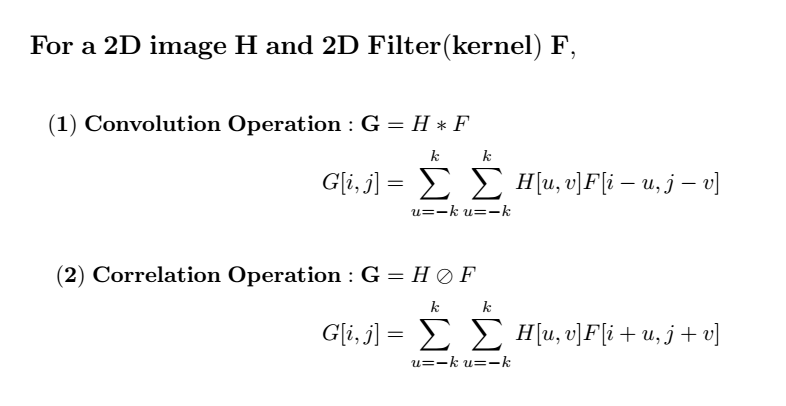# [DL] 8. CNN 1(Convolutional Neural Network Basics)

Original article can be found here (source): Deep Learning on Medium# 1. Convolution Operation

In mathematics, the convolution is a mathematical operation on two functions(f and g) that produces a third function expressing how the shape of one is modified by the other.

In other words, convolution is one way of applying a filter to one function and obtaining the resulting function. The concept of convolution operation is widely used over the various fields of computer science.

In audio processing, the original function is an audio signal(1D or higher) and we apply the filter(1D or higher) using convolution.

In computer vision, as we are dealing with images, the original function is a 2D image and the filter we want to apply is also 2 dimensional.

Then, let’s take a look at how the convolution operation looks like.

According to our intuition, applying a filter to an image is supposed to look like the second operation, the correlation. In practice, however, the convolution operation is as written in equation (1).

The meaning of the negative sign in F[i-u, j-v] is that first we flip the filter F[u,v] into F[-u,-v] and translate it by i and j so that eventually the filter F[u,v] becomes F[i-u, j-v]. And finally perform the multiplication with the image H[u,v] to get the resulting value G[i,j]

• Convolution vs Correlation

The figure 2 shows the difference between convolution and correlation operation.

# 2. Convolution in images

The below figure represents the way of how does the convolution works with images.

The left-most column is the input image of size 7×7 with three channels, the next to columns are the two filters we apply to the input image and the right-most column is the resulting output of convolution(Input image, Filter).

Note that the filter W has the same number of channels as the input image has since elements in each channel of an input image are multiplied with filter weights from respective channels of the filter and those intermediate results are being summed to output the result of convolution.

In figure 3, the elements of an input image in blue box are element-wise multiplied with filter weights in red box to make intermediate results, and then those results are being summed to make the final output ‘-10’ which is in the green box.

## (1) Local receptive fields

The definition of receptive fields is that the area in input where is being multiplied by the filter. For example, the area surrounded by the blue box of the input image in figure 3 is an example of a receptive field.

The size of this area is decided by the size of the filter(also called as a kernel), meaning that we can process images with varying sizes of the filter that we want to apply unless the filter size doesn’t exceed the input size.

## (2) Sparse Connectivity

In the case of the FC(fully connected) layer, all hidden units in layer (L) is connected to one hidden unit in the next layer (L+1). This means that if there are 5 hidden units in each layer as is shown in figure 4, then 25 weights are required in total. For example, all hidden unit x1~x5 are contributing to one hidden unit s3 of the layer (L+1). In order to produce this relation, we need 5 weights. And there are 4 more hidden units in (L+1). Therefore, we need 25 weights in total.

In layers connected by convolution, however, only need 3 weights to produce s3 as only three hidden units x2, x3, x4 are contributing to s3. Compared to the s3 with FC layer, the s3 with convolution has fewer connections in the hidden units of the previous layer (L), and this means it has a sparser connection than FC.

We have seen that convolution requires less number of weights than FC but one might ask that the s3 with convolution may lose the information from x1 and x5 whereas s3 with FC has information from all hidden units in the previous layer.

Actually this issue can be solved by stacking layers of convolution as figure 5 shows.

In this figure, the unit y3 indirectly interacts with all hidden units x1 ~ x5 through h2~h4 showing that the information of x1~x5 also flows to y3.

## (3) Parameter sharing

In FC layer, each weight is used only once when computing the activation for subsequent layers. In a CNN, however, the weights of each filter are applied to every position of the input, meaning that each weight is used more than once and is shared.

Since the weight parameters are shared, it requires less memory space than FC and we can stack more layers in our network.

In CNN, lines(weights) marked with the same color share their value. In other words, there are only 3 weights with different values. In FC, on the other hand, each weight has its own value and doesn’t share its value with any other weights, meaning there are 25(5ⅹ5) weights for the reason explained before.

## (4) equivariant representation & translation-invariant

As a same filter travels over the whole input image and outputs the result of convolution, if that filter is capable of detecting a certain object and that object repeatedly appears in the image, we can detect all of those by convolution.

More specifically, by training the CNN the filter becomes capable of finding certain patterns, and since that filter is applied to every subregion of the input image, it can find all appearance of such pattern regardless of how often that pattern appears in the input image. And this is why the filter of CNN is called translation-invariant.

# 4. Reference

 Bishop. Pattern Recognition and Machine Learning. Springer, 2006

 GoodFellow

 CS231

Any corrections, suggestions, and comments are welcome